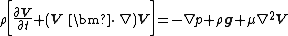Navier-Stokes equation

Navier-Stokes equation

A partial differential equation which describes the conservation of linear momentum for a linearly viscous (newtonian), incompressible fluid flow. In vector form, this relation is written as Eq. (1),

(1)where &rgr; is fluid density, V is fluid velocity, p is fluid pressure, g is the gravitational acceleration, μ is fluid viscosity, ∇ is the del or grad operator, and ∇2 is the laplacian operator. The equation is named after its two principal developers, French engineer C. L. M. H. Navier (1823) and Irish scientist George G. Stokes (1845). When coupled with the conservation of mass relation, ∇ · V = 0, Eq. (1) can be solved for the space-time distribution of V and p in a given region of viscous fluid flow. Typical boundary conditions are (1) the knowledge of the velocity and pressure in the far field, and (2) the no-slip condition at solid surfaces (fluid velocity equals solid velocity). See Newtonian fluid, Viscosity

Equation (1) correctly models the continuum behavior of all newtonian fluids, including the disorderly fluctuating motion known as turbulence. However, since the left-hand side is highly nonlinear, only a few score of exact solutions are known, mostly for simple geometries. The primary dimensionless parameter which governs Eq. (1) is the Reynolds number, given by Eq. (2),

(2)where L is a characteristic body dimension. For small Re 1, Eq. (1) can be simplified by neglecting the left-hand side, resulting in a linear approximation called Stokes flow, or creeping flow, for which many solutions are known. See Creeping flow, Reynolds number

For large Re 1, viscous effects are often confined to a thin boundary layer near solid surfaces, with the remaining flow being nearly inviscid. See Boundary-layer flow

McGraw-Hill Concise Encyclopedia of Physics. © 2002 by The McGraw-Hill Companies, Inc.
References in periodicals archive ?
The strain rate from Navier-Stokes equation is approximately 49 percent greater than that from Reynolds theory at step tip and too strong to be omitted.
A fully correct transport (FCT) method was used to solve the full compressible Navier-Stokes equation. Flow patterns were shown to strongly correlate to the rapidity of the wall heating process.
Internal waves are generated through the interaction between the tidal flow and the topography in a nonuniform fluid layer by solving the Navier-Stokes equation in Boussinesq approximation.
 proposed a generalized Navier-Stokes equation for isothermal incompressible flow in porous media, which ignores the detailed structure of the porous media by including an additional term to account for the presence of a porous medium.
High-resolutions for incompressible Navier-Stokes equation and a multi-grid method, J.
The NWF can simulate a free-surface flow, the governing equation of which is Navier-Stokes equation. In case of an incompressible Newtonian viscous fluid, continuity equation and Navier-Stokes equation are as follows:
The numerical solution of the viscous flow problem for material flow in the 2[theta] angular ECAE die AOC-BED requires the formulation of a boundary value problem (Appendix A) for the Navier-Stokes equation in the curl (vorticity) transfer form (1)-(2) for [mathematical expression not reproducible], where [zeta] = [partial derivative]u/[partial derivative]y - [partial derivative]v/[partial derivative]x (Figures 6(c), 6(d), 7(c), 7(d), 8(c), and 8(d)).
The solution of the fluid side is based on the continuity equation and the Navier-Stokes equation. The Navier-Stokes equation, written in Einstein summation convention, is given by (1):
Non-Newtonian fluids are the most widely occurring fluids in industry and engineering and its governing equations are highly nonlinear as compared to the Navier-Stokes equation. Non-Newtonian fluids are complex in nature because their rheological properties are involved as "parameters" in the constitutive equation.
Soltanov: On some modification Navier-Stokes equation, Nonlinear Anal., 52(2003), No.
Fully nonlinear potential flow (FNPF) and incompressible Navier-Stokes equation are common governing equations that are used for simulation of the periodic wave interactions in the coastal zone.

Site: Follow: Share:
Open / Close